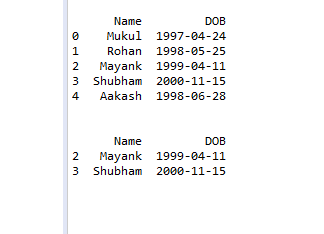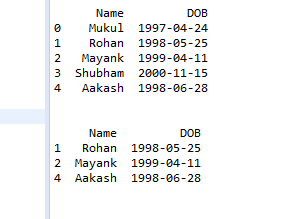Related Articles

# Filter Pandas DataFrame by Time

• Last Updated : 24 Feb, 2021

In this article let’s see how to filter pandas data frame by date. So we can filter python pandas data frame by date using the logical operator and loc() method. In the below examples we have a data frame that contains two columns the first column is Name and another one is DOB.

Example 1: filter data that’s DOB is greater than 1999-02-5.

## Python

 `import` `pandas as pd`` ` `# create data frame``Data ``=` `{``'Name'``: [``'Mukul'``, ``'Rohan'``, ``'Mayank'``,``                          ``'Shubham'``, ``'Aakash'``],`` ` `        ``'DOB'``: [``'1997-04-24'``, ``'1998-05-25'``, ``'1999-04-11'``,``                ``'2000-11-15'``, ``'1998-06-28'``]}`` ` `df ``=` `pd.DataFrame(Data)`` ` `# print original data frame``print``(df)`` ` `# filter data frame``New_df ``=` `df.loc[df[``"DOB"``] >``=` `"1999-02-5"``]`` ` `# print filtered data frame``print``(New_df)`

Output:Example 2: filter data between two date.

## Python

 `import` `pandas as pd`` ` `# create data frame``Data ``=` `{``'Name'``: [``'Mukul'``, ``'Rohan'``, ``'Mayank'``,``                          ``'Shubham'``, ``'Aakash'``],`` ` `        ``'DOB'``: [``'1997-04-24'``, ``'1998-05-25'``, ``'1999-04-11'``,``                ``'2000-11-15'``, ``'1998-06-28'``]}``df ``=` `pd.DataFrame(Data)`` ` `# print original data frame``print``(df)`` ` `Date1 ``=` `df[``"DOB"``] >``=` `"1998-04-24"``Date2 ``=` `df[``"DOB"``] <``=` `"1999-1-31"`` ` `# filter data between 1998-04-24 to 1999-01-31``New_df ``=` `df.loc[Date1 & Date2]`` ` `# print the filtered data frame``print``(New_df)`

Output:Attention geek! Strengthen your foundations with the Python Programming Foundation Course and learn the basics.

To begin with, your interview preparations Enhance your Data Structures concepts with the Python DS Course. And to begin with your Machine Learning Journey, join the Machine Learning – Basic Level Course

My Personal Notes arrow_drop_up